# BJT common emitter amplifier - Distortion of output signal wave and finding the upper -3dB frequency

#### hope estheim

Joined May 20, 2017
9
Hi,
I was suppose to construct this circuit using Multisim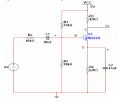where Vs is sinusoidal signal 0.1Vpeak, 10kHz.
This is my simulation circuit from Multisim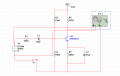This is the result of oscilloscope for both signal waveform, Vs and Vc.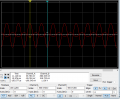As you can see from the oscilloscope, the output voltage is amplified, with the voltage gain around -5.2 V/V

The problem is, if I increase the amplitude of the input signal source,for example from Vp=0.1 to Vp=0.5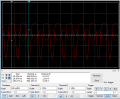It will have this weird distortion, and the output wave seems to have shift down.Moreover the gain is reduced!
I have research about the BJT again. In my opinion, I think this is because the bias voltage has changed and thus the Q point also changes so it lies in cut off mode.
However , I thought the clipping will occur if the output is greater than the limit supply voltage rail which is Vcc=5V.but it clips at +1.024V.
Do I have to look at DCload line to find out the limit?

if I further increase the amplitude, the gain reduces more but the clipping still remains at around+1.90V and the negative part of the output wave also starts to clip.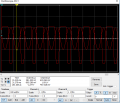To find the upper -3db frequency, I have to find the bandwidth of amplifier.
So what I do is,
take the voltage gain(-5.2V/V) multiply by 0.707
which is -3.6764V/V
Then using the new voltage gain, multiply by the 0.1Vp
which is -0.36764V.
So my output waveform should get max peak -0.36744 V right?then just check the period for the wave,then proceed to find frequency

But If I change the frequency on the ac voltage source, the output remain same just the wave is stretched out.
If I try to use bode plotter,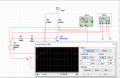The straight constant line is not there?so I cant get the bandwidth.

Any inputs or comment for this matter is appreciated.Please correct me if I have stated the wrong theory or if there is something wrong for my circuit
Thanks again for your help and patience.

#### Jony130

Joined Feb 17, 2009
5,241
What is the DC voltage at emitter and collector? And do you know anything about saturation? Because the clipping occurs when the BJT enters saturation or cut-off region.

#### hope estheim

Joined May 20, 2017
9
What is the DC voltage at emitter and collector? And do you know anything about saturation? Because the clipping occurs when the BJT enters saturation or cut-off region.
I just probe the voltage and i get these value
V emitter=1.30V
V collector= 4.02V
V base= 1.94V

To be frank I just start to study about BJT today, so not quite familiar about saturation as well.
How do you know at what point it gets saturation? Is it when I collector went to max?

#### shteii01

Joined Feb 19, 2010
4,644
The distortion looks like you are hitting upper rail. So I guess your dc bias is in the wrong place/level.

Pro tip: on the schematic right click on the wire, choose color, change color to something other than red. This way when you do oscilloscope plot, you will have two signals and each signal will be different color.

#### hope estheim

Joined May 20, 2017
9
The distortion looks like you are hitting upper rail. So I guess your dc bias is in the wrong place/level.

Pro tip: on the schematic right click on the wire, choose color, change color to something other than red. This way when you do oscilloscope plot, you will have two signals and each signal will be different color.
Hi, thanks for the tip, i have change the wire color and it is a lot more easier interpreting the wave!
What do you mean by the the dc bias is in the wrong place?Care to elaborate more?Why is it clipped at 1.092 V specifically?

#### shteii01

Joined Feb 19, 2010
4,644
Hi, thanks for the tip, i have change the wire color and it is a lot more easier interpreting the wave!
What do you mean by the the dc bias is in the wrong place?Care to elaborate more?Why is it clipped at 1.092 V specifically?

The basic thing to remember is that dc bias (or maybe dc line is better) provides the line/level. AC voltage oscillates around this line/level.
Notice that your oscilloscope is AC coupled. This means that dc bias is blocked. That is why both ac signals are oscillating around 0V line. And everything looks so nice and pretty.
The reality might be something else.
Your first plot, where input and output signals are very nice clean sine waves is obviously have dc bias in the right place. Your full range is from 0 to 5V. Your input signal is about 100 mV peak, you output signal is about 600 mV peak. I did not do the math so I don't know where your dc bias is, probably around 2 or 2.5 volts, which puts you in the middle of the 5-0=5V range. Your rails here are 0V and 5V, and you are nowhere near it. Again. Assuming your dc bias is at the 2.5V. Then your input signal is sine wave from 2.4V to 2.6V. Your output signal is from 1.9V to 3.1V. Select the dc coupling and see what the circuit is doing.

•hope estheim

#### hope estheim

Joined May 20, 2017
9

The basic thing to remember is that dc bias (or maybe dc line is better) provides the line/level. AC voltage oscillates around this line/level.
Notice that your oscilloscope is AC coupled. This means that dc bias is blocked. That is why both ac signals are oscillating around 0V line. And everything looks so nice and pretty.
The reality might be something else.
Your first plot, where input and output signals are very nice clean sine waves is obviously have dc bias in the right place. Your full range is from 0 to 5V. Your input signal is about 100 mV peak, you output signal is about 600 mV peak. I did not do the math so I don't know where your dc bias is, probably around 2 or 2.5 volts, which puts you in the middle of the 5-0=5V range. Your rails here are 0V and 5V, and you are nowhere near it. Again. Assuming your dc bias is at the 2.5V. Then your input signal is sine wave from 2.4V to 2.6V. Your output signal is from 1.9V to 3.1V. Select the dc coupling and see what the circuit is doing.
Wow, after I put DC coupling for both waveform, I found at that it really clipped at 5V , which is the limit of supply rails! Now I understand because I keep on wondering why it is not at 5V.
If i increase the amplitude of the voltage signal much more,the bottom clipping occurs.If you don't mind me asking , how we find out the at what voltage the bottom clipping occurs?

#### hope estheim

Joined May 20, 2017
9
For Vc = 4V the collector voltage can only swing up be 1V before reaching Vcc limit. And about 2V down before saturation occurred.

If you have the time try to read this
the illustration about BJT from the first link is very straightforward and easy to understand!
But why did you say it swings down 2V before it goes to saturation?How did you deduce the 2V?

#### Jony130

Joined Feb 17, 2009
5,241
But why did you say it swings down 2V before it goes to saturation?How did you deduce the 2V?
At first glance we can safely estimate this (Vc - Vb = 4V - 2V = 2V) Or Vc - Ve+Vce(sat) = Vc - Ve+0.2VNotice that for Vin = 1V the voltage at emitter also swings by 1V (from 2V to 3V and from 2V down to 1V).
And I hope that you can see that Vout_max = 12V but Vout_(min) = 3V. Because any input voltage larger than 1V will cause BJT to saturate and clipping (Ve - want to be larger than 3V, but at the same time Vc want to be smaller than 3V).
So for this circuit V(max) = 12V and V(min) = 3V = 12V*Re/(Re+Rc) for Vce(sat) = 0V.

•hope estheim

#### hope estheim

Joined May 20, 2017
9
At first glance we can safely estimate this (Vc - Vb = 4V - 2V = 2V) Or Vc - Ve+Vce(sat) = Vc - Ve+0.2V

View attachment 127269

Notice that for Vin = 1V the voltage at emitter also swings by 1V (from 2V to 3V and from 2V down to 1V).
And I hope that you can see that Vout_max = 12V but Vout_(min) = 3V. Because any input voltage larger than 1V will cause BJT to saturate and clipping (Ve - want to be larger than 3V, but at the same time Vc want to be smaller than 3V).
So for this circuit V(max) = 12V and V(min) = 3V = 12V*Re/(Re+Rc) for Vce(sat) = 0V.
Sir,
I don't know if saying a million times of thanks would be enough.
I finally get it.This is actually my lab question where it asks us to explain why the distortion occurs, and before going into the lab details, i have zero idea on the BJT and staturation point as I could not understand what the lecturer is trying to explain.
Thanks again sir!

#### hope estheim

Joined May 20, 2017
9
How do I find the cutoff frequency in multisim??

#### MrAl

Joined Jun 17, 2014
8,385
How do I find the cutoff frequency in multisim??
Hi,

Do you know what it means to see a gain that is 3db down (-3db) from the passband gain?

#### hope estheim

Joined May 20, 2017
9
Hi,

Do you know what it means to see a gain that is 3db down (-3db) from the passband gain?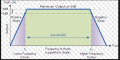Is it something like this?
I use the bode plotter but it shows the graph like this
Voltage gain at -3dB = Voltage gain initial x 0.707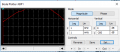Is it similar

#### MrAl

Joined Jun 17, 2014
8,385
Hi,

Yes, that's it. You use the highest value in the passband as the base gain value.
I think you know how to do this now?
You test for that -3db point(s) using the simulator.

#### hope estheim

Joined May 20, 2017
9
Hi,

Yes, that's it. You use the highest value in the passband as the base gain value.
I think you know how to do this now?
You test for that -3db point(s) using the simulator.
Hi,
I set the Y- value to -3dB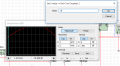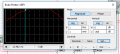is 13.462Hz the lower cut off frequency?

#### Jony130

Joined Feb 17, 2009
5,241
For Ve = 1.3V we have Ie = 1.3V/620Ω = 2mA and if the BJT current gain is around β = 150 we can find

rpi≈ 150*(26mV/2mA) ≈ 1.95kΩ

F1 ≈ 0.16/(Rth1*C1)

Where Rth1 is a equivale resistance seen by C1 capacitor.

Rth1 = Rs+(R1||R2||rpi) = 10kΩ+(15kΩ||10kΩ||1.95kΩ) ≈ 11.5kΩ

F1 ≈ 0.16/(Rth1*C1) ≈ 1.39Hz

And F2 ≈ 0.16/(Rth2*C2)

Rth2 = (Rs||R1||R2)/(β+1) + re = 24.9Ω + 26mV/2mA ≈ 38Ω

F2 ≈ 0.16/(Rth2*C2) ≈ 8.96Hz

#### MrAl

Joined Jun 17, 2014
8,385
Hi,
I set the Y- value to -3dB
View attachment 127289
View attachment 127290
is 13.462Hz the lower cut off frequency?
Hi,

Well the thing that bothers me is we cant see the maximum point on your curve. We need to see that, and we need to see the scale of the Y axis. We need to be able to see the maximum point on the wave to see where the -3db point is. For example, if the peak was at 20db then the -3db ponit would be at 17db. So if you set a straight line across the graph at 17db you would see where it intersects the curve and that would show you the two -3 db points graphically. If the max point on the curve was 12db then the -3db point would be at 9db. It's that simple, but we must see the highest point on the curve in the graph, and the Y axis scale must show the labels such as 1db, 2db, 3db, etc.
There are times when we dont use the actual maximum as the reference, when we have wildly varying curves, and then we look for the point where we consider the response to be in the passband, then take the points 3db lower than that as the -3db points.